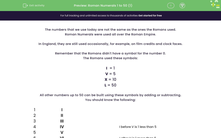# Understand Roman Numerals 1 to 50

In this worksheet, students will convert Roman numerals to normal numbers up to 50.Key stage:  KS 2

Curriculum topic:   Number: Number and Place Value

Curriculum subtopic:   Read Roman Numerals (I to C/1 to 100)

Difficulty level:#### Worksheet Overview

The numbers that we use today are not the same as the ones the Romans used.

Roman numerals were used all over the Roman Empire.In the United Kingdom, they are still used occasionally, for example, on film credits and clock faces.

Remember that the Romans didn't have a symbol for the number 0.

The Romans used these symbols:

I  = 1

V = 5

X = 10

L = 50

All other numbers up to 50 can be built using these symbols by adding or subtracting.

You should know the following:

 1 I 2 II 3 III 4 IV I before V is 1 less than 5 5 V 6 VI I after V is 1 more than 5 7 VII II after V is 2 more than 5 8 VIII III after V is 3 more than 5 9 IX I before X is 1 less than 10 10 X 11 XI I after X is 1 more than 10 12 XII II after X is 2 more than 10

We build bigger numbers by adding 10s or Xs in Roman numerals.

 20 XX = X + X = 20 21 XXI = XX + I = 20 + 1 30 XXX = X + X + X = 30 37 XXXVII = XXX + VII = 30 + 7 40 XL = X before L is 10 less than 50 or 50 - 10 = 40 48 XLVIII = XL + VIII = 40 + 8 = 48 49 XLIX = XL + IX = 40 + 9 = 49 50 L

Example

Convert XLIV to a normal number.

XLIV = XL + IV = 40 + 4 = 44

Are you happy to get started now?### What is EdPlace?

We're your National Curriculum aligned online education content provider helping each child succeed in English, maths and science from year 1 to GCSE. With an EdPlace account you’ll be able to track and measure progress, helping each child achieve their best. We build confidence and attainment by personalising each child’s learning at a level that suits them.

Get started